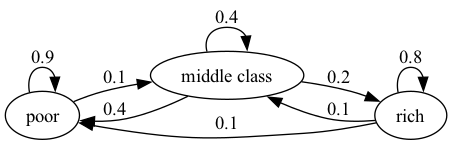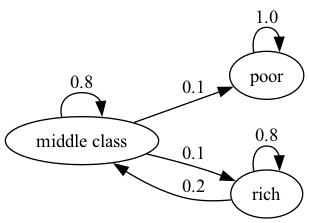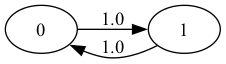# 26. Markov Chains: Irreducibility and Ergodicity#

In addition to what’s in Anaconda, this lecture will need the following libraries:

!pip install quantecon

Hide code cell output
Requirement already satisfied: quantecon in /usr/share/miniconda3/envs/quantecon/lib/python3.10/site-packages (0.7.1)

Requirement already satisfied: numba>=0.49.0 in /usr/share/miniconda3/envs/quantecon/lib/python3.10/site-packages (from quantecon) (0.56.4)
Requirement already satisfied: requests in /usr/share/miniconda3/envs/quantecon/lib/python3.10/site-packages (from quantecon) (2.31.0)
Requirement already satisfied: scipy>=1.5.0 in /usr/share/miniconda3/envs/quantecon/lib/python3.10/site-packages (from quantecon) (1.10.0)
Requirement already satisfied: numpy>=1.17.0 in /usr/share/miniconda3/envs/quantecon/lib/python3.10/site-packages (from quantecon) (1.23.5)
Requirement already satisfied: sympy in /usr/share/miniconda3/envs/quantecon/lib/python3.10/site-packages (from quantecon) (1.11.1)
Requirement already satisfied: setuptools in /usr/share/miniconda3/envs/quantecon/lib/python3.10/site-packages (from numba>=0.49.0->quantecon) (65.6.3)
Requirement already satisfied: llvmlite<0.40,>=0.39.0dev0 in /usr/share/miniconda3/envs/quantecon/lib/python3.10/site-packages (from numba>=0.49.0->quantecon) (0.39.1)
Requirement already satisfied: certifi>=2017.4.17 in /usr/share/miniconda3/envs/quantecon/lib/python3.10/site-packages (from requests->quantecon) (2022.12.7)
Requirement already satisfied: urllib3<3,>=1.21.1 in /usr/share/miniconda3/envs/quantecon/lib/python3.10/site-packages (from requests->quantecon) (1.26.14)
Requirement already satisfied: charset-normalizer<4,>=2 in /usr/share/miniconda3/envs/quantecon/lib/python3.10/site-packages (from requests->quantecon) (2.0.4)
Requirement already satisfied: idna<4,>=2.5 in /usr/share/miniconda3/envs/quantecon/lib/python3.10/site-packages (from requests->quantecon) (3.4)
Requirement already satisfied: mpmath>=0.19 in /usr/share/miniconda3/envs/quantecon/lib/python3.10/site-packages/mpmath-1.2.1-py3.10.egg (from sympy->quantecon) (1.2.1)


## 26.1. Overview#

This lecture continues on from our earlier lecture on Markov chains.

Specifically, we will introduce the concepts of irreducibility and ergodicity, and see how they connect to stationarity.

Irreducibility describes the ability of a Markov chain to move between any two states in the system.

Ergodicity is a sample path property that describes the behavior of the system over long periods of time.

As we will see,

• an irreducible Markov chain guarantees the existence of a unique stationary distribution, while

• an ergodic Markov chain generates time series that satisfy a version of the law of large numbers.

Together, these concepts provide a foundation for understanding the long-term behavior of Markov chains.

import matplotlib.pyplot as plt
plt.rcParams["figure.figsize"] = (11, 5)  # set default figure size
import quantecon as qe
import numpy as np
import networkx as nx
from matplotlib import cm
import matplotlib as mpl


## 26.2. Irreducibility#

To explain irreducibility, let’s take $$P$$ to be a fixed stochastic matrix.

Two states $$x$$ and $$y$$ are said to communicate with each other if there exist positive integers $$j$$ and $$k$$ such that

$P^j(x, y) > 0 \quad \text{and} \quad P^k(y, x) > 0$

In view of our discussion above, this means precisely that

• state $$x$$ can eventually be reached from state $$y$$, and

• state $$y$$ can eventually be reached from state $$x$$

The stochastic matrix $$P$$ is called irreducible if all states communicate; that is, if $$x$$ and $$y$$ communicate for all $$(x, y)$$ in $$S \times S$$.

For example, consider the following transition probabilities for wealth of a fictitious set of householdsWe can translate this into a stochastic matrix, putting zeros where there’s no edge between nodes

$\begin{split} P := \begin{bmatrix} 0.9 & 0.1 & 0 \\ 0.4 & 0.4 & 0.2 \\ 0.1 & 0.1 & 0.8 \end{bmatrix} \end{split}$

It’s clear from the graph that this stochastic matrix is irreducible: we can eventually reach any state from any other state.

We can also test this using QuantEcon.py’s MarkovChain class

P = [[0.9, 0.1, 0.0],
[0.4, 0.4, 0.2],
[0.1, 0.1, 0.8]]

mc = qe.MarkovChain(P, ('poor', 'middle', 'rich'))
mc.is_irreducible

True


Here’s a more pessimistic scenario in which poor people remain poor foreverThis stochastic matrix is not irreducible since, for example, rich is not accessible from poor.

Let’s confirm this

P = [[1.0, 0.0, 0.0],
[0.1, 0.8, 0.1],
[0.0, 0.2, 0.8]]

mc = qe.MarkovChain(P, ('poor', 'middle', 'rich'))
mc.is_irreducible

False


It might be clear to you already that irreducibility is going to be important in terms of long-run outcomes.

For example, poverty is a life sentence in the second graph but not the first.

We’ll come back to this a bit later.

### 26.2.1. Irreducibility and stationarity#

We discussed the uniqueness of the stationary in the previous lecture requires the transition matrix to be everywhere positive.

In fact irreducibility is enough for the uniqueness of the stationary distribution to hold if the distribution exists.

We can revise the theorem into the following fundamental theorem:

Theorem 26.1

If $$P$$ is irreducible, then $$P$$ has exactly one stationary distribution.

For proof, see Chapter 4 of [SS23] or Theorem 5.2 of .

## 26.3. Ergodicity#

Please note that we use $$\mathbb{1}$$ for a vector of ones in this lecture.

Under irreducibility, yet another important result obtains:

Theorem 26.2

If $$P$$ is irreducible and $$\psi^*$$ is the unique stationary distribution, then, for all $$x \in S$$,

(26.1)#$\frac{1}{m} \sum_{t = 1}^m \mathbf{1}\{X_t = x\} \to \psi^*(x) \quad \text{as } m \to \infty$

Here

• $$\{X_t\}$$ is a Markov chain with stochastic matrix $$P$$ and initial. distribution $$\psi_0$$

• $$\mathbb{1} \{X_t = x\} = 1$$ if $$X_t = x$$ and zero otherwise.

The result in (26.1) is sometimes called ergodicity.

The theorem tells us that the fraction of time the chain spends at state $$x$$ converges to $$\psi^*(x)$$ as time goes to infinity.

This gives us another way to interpret the stationary distribution (provided irreducibility holds).

Importantly, the result is valid for any choice of $$\psi_0$$.

The theorem is related to the Law of Large Numbers.

It tells us that, in some settings, the law of large numbers sometimes holds even when the sequence of random variables is not IID.

### 26.3.1. Example 1#

Recall our cross-sectional interpretation of the employment/unemployment model discussed above.

Assume that $$\alpha \in (0,1)$$ and $$\beta \in (0,1)$$, so that irreducibility holds.

We saw that the stationary distribution is $$(p, 1-p)$$, where

$p = \frac{\beta}{\alpha + \beta}$

In the cross-sectional interpretation, this is the fraction of people unemployed.

In view of our latest (ergodicity) result, it is also the fraction of time that a single worker can expect to spend unemployed.

Thus, in the long run, cross-sectional averages for a population and time-series averages for a given person coincide.

This is one aspect of the concept of ergodicity.

### 26.3.2. Example 2#

Another example is the Hamilton dynamics we discussed before.

The graph of the Markov chain shows it is irreducible

Therefore, we can see the sample path averages for each state (the fraction of time spent in each state) converges to the stationary distribution regardless of the starting state

Let’s denote the fraction of time spent in state $$x$$ at time $$t$$ in our sample path as $$\hat p_t(x)$$ where

$\hat p_t(x) := \frac{1}{t} \sum_{t = 1}^t \mathbf{1}\{X_t = x\}$

Here we compare $$\hat p_t(x)$$ with the stationary distribution $$\psi^* (x)$$ for different starting points $$x_0$$.

P = np.array([[0.971, 0.029, 0.000],
[0.145, 0.778, 0.077],
[0.000, 0.508, 0.492]])
ts_length = 10_000
mc = qe.MarkovChain(P)
n = len(P)
fig, axes = plt.subplots(nrows=1, ncols=n, figsize=(15, 6))
ψ_star = mc.stationary_distributions

for i in range(n):
axes[i].axhline(ψ_star[i], linestyle='dashed', lw=2, color='black',
label = fr'$\psi^*({i})$')
axes[i].set_xlabel('t')
axes[i].set_ylabel(fr'$\hat p_t({i})$')

# Compute the fraction of time spent, starting from different x_0s
for x0, col in ((0, 'blue'), (1, 'green'), (2, 'red')):
# Generate time series that starts at different x0
X = mc.simulate(ts_length, init=x0)
p_hat = (X == i).cumsum() / (1 + np.arange(ts_length, dtype=float))
axes[i].plot(p_hat, color=col, label=f'$x_0 = \, {x0}$')
axes[i].legend()
plt.show()


Note the convergence to the stationary distribution regardless of the starting point $$x_0$$.

### 26.3.3. Example 3#

Let’s look at one more example with six states discussed before.

$\begin{split} P := \begin{bmatrix} 0.86 & 0.11 & 0.03 & 0.00 & 0.00 & 0.00 \\ 0.52 & 0.33 & 0.13 & 0.02 & 0.00 & 0.00 \\ 0.12 & 0.03 & 0.70 & 0.11 & 0.03 & 0.01 \\ 0.13 & 0.02 & 0.35 & 0.36 & 0.10 & 0.04 \\ 0.00 & 0.00 & 0.09 & 0.11 & 0.55 & 0.25 \\ 0.00 & 0.00 & 0.09 & 0.15 & 0.26 & 0.50 \end{bmatrix} \end{split}$

The graph for the chain shows all states are reachable, indicating that this chain is irreducible.

Here we visualize the difference between $$\hat p_t(x)$$ and the stationary distribution $$\psi^* (x)$$ for each state $$x$$

P = [[0.86, 0.11, 0.03, 0.00, 0.00, 0.00],
[0.52, 0.33, 0.13, 0.02, 0.00, 0.00],
[0.12, 0.03, 0.70, 0.11, 0.03, 0.01],
[0.13, 0.02, 0.35, 0.36, 0.10, 0.04],
[0.00, 0.00, 0.09, 0.11, 0.55, 0.25],
[0.00, 0.00, 0.09, 0.15, 0.26, 0.50]]

ts_length = 10_000
mc = qe.MarkovChain(P)
ψ_star = mc.stationary_distributions
fig, ax = plt.subplots(figsize=(9, 6))
X = mc.simulate(ts_length)
# Center the plot at 0
ax.set_ylim(-0.25, 0.25)
ax.axhline(0, linestyle='dashed', lw=2, color='black', alpha=0.4)

for x0 in range(6):
# Calculate the fraction of time for each state
p_hat = (X == x0).cumsum() / (1 + np.arange(ts_length, dtype=float))
ax.plot(p_hat - ψ_star[x0], label=f'$x = {x0+1}$')
ax.set_xlabel('t')
ax.set_ylabel(r'$\hat p_t(x) - \psi^* (x)$')

ax.legend()
plt.show()


Similar to previous examples, the sample path averages for each state converge to the stationary distribution.

### 26.3.4. Example 4#

Let’s look at another example with two states: 0 and 1.

$\begin{split} P := \begin{bmatrix} 0 & 1\\ 1 & 0\\ \end{bmatrix} \end{split}$

The diagram of the Markov chain shows that it is irreducibleIn fact it has a periodic cycle — the state cycles between the two states in a regular way.

This is called periodicity.

It is still irreducible so ergodicity holds.

P = np.array([[0, 1],
[1, 0]])
ts_length = 10_000
mc = qe.MarkovChain(P)
n = len(P)
fig, axes = plt.subplots(nrows=1, ncols=n)
ψ_star = mc.stationary_distributions

for i in range(n):
axes[i].set_ylim(0.45, 0.55)
axes[i].axhline(ψ_star[i], linestyle='dashed', lw=2, color='black',
label = fr'$\psi^*({i})$')
axes[i].set_xlabel('t')
axes[i].set_ylabel(fr'$\hat p_t({i})$')

# Compute the fraction of time spent, for each x
for x0 in range(n):
# Generate time series starting at different x_0
X = mc.simulate(ts_length, init=x0)
p_hat = (X == i).cumsum() / (1 + np.arange(ts_length, dtype=float))
axes[i].plot(p_hat, label=f'$x_0 = \, {x0}$')

axes[i].legend()
plt.show()


This example helps to emphasize that asymptotic stationarity is about the distribution, while ergodicity is about the sample path.

The proportion of time spent in a state can converge to the stationary distribution with periodic chains.

However, the distribution at each state does not.

### 26.3.5. Expectations of geometric sums#

Sometimes we want to compute the mathematical expectation of a geometric sum, such as $$\sum_t \beta^t h(X_t)$$.

In view of the preceding discussion, this is

$\mathbb{E} \left[ \sum_{j=0}^\infty \beta^j h(X_{t+j}) \mid X_t = x \right] = x + \beta (Ph)(x) + \beta^2 (P^2 h)(x) + \cdots$

By the Neumann series lemma, this sum can be calculated using

$I + \beta P + \beta^2 P^2 + \cdots = (I - \beta P)^{-1}$

## 26.4. Exercises#

Exercise 26.1

Benhabib el al. [BBL19] estimated that the transition matrix for social mobility as the following

$\begin{split} P:= \begin{bmatrix} 0.222 & 0.222 & 0.215 & 0.187 & 0.081 & 0.038 & 0.029 & 0.006 \\ 0.221 & 0.22 & 0.215 & 0.188 & 0.082 & 0.039 & 0.029 & 0.006 \\ 0.207 & 0.209 & 0.21 & 0.194 & 0.09 & 0.046 & 0.036 & 0.008 \\ 0.198 & 0.201 & 0.207 & 0.198 & 0.095 & 0.052 & 0.04 & 0.009 \\ 0.175 & 0.178 & 0.197 & 0.207 & 0.11 & 0.067 & 0.054 & 0.012 \\ 0.182 & 0.184 & 0.2 & 0.205 & 0.106 & 0.062 & 0.05 & 0.011 \\ 0.123 & 0.125 & 0.166 & 0.216 & 0.141 & 0.114 & 0.094 & 0.021 \\ 0.084 & 0.084 & 0.142 & 0.228 & 0.17 & 0.143 & 0.121 & 0.028 \end{bmatrix} \end{split}$

where each state 1 to 8 corresponds to a percentile of wealth shares

$0-20 \%, 20-40 \%, 40-60 \%, 60-80 \%, 80-90 \%, 90-95 \%, 95-99 \%, 99-100 \%$

The matrix is recorded as P below

P = [
[0.222, 0.222, 0.215, 0.187, 0.081, 0.038, 0.029, 0.006],
[0.221, 0.22,  0.215, 0.188, 0.082, 0.039, 0.029, 0.006],
[0.207, 0.209, 0.21,  0.194, 0.09,  0.046, 0.036, 0.008],
[0.198, 0.201, 0.207, 0.198, 0.095, 0.052, 0.04,  0.009],
[0.175, 0.178, 0.197, 0.207, 0.11,  0.067, 0.054, 0.012],
[0.182, 0.184, 0.2,   0.205, 0.106, 0.062, 0.05,  0.011],
[0.123, 0.125, 0.166, 0.216, 0.141, 0.114, 0.094, 0.021],
[0.084, 0.084, 0.142, 0.228, 0.17,  0.143, 0.121, 0.028]
]

P = np.array(P)
codes_B = ('1','2','3','4','5','6','7','8')


In this exercise,

1. show this process is asymptotically stationary and calculate the stationary distribution using simulations.

2. use simulations to demonstrate ergodicity of this process.

Exercise 26.2

According to the discussion above, if a worker’s employment dynamics obey the stochastic matrix

$\begin{split} P := \begin{bmatrix} 1 - \alpha & \alpha \\ \beta & 1 - \beta \end{bmatrix} \end{split}$

with $$\alpha \in (0,1)$$ and $$\beta \in (0,1)$$, then, in the long run, the fraction of time spent unemployed will be

$p := \frac{\beta}{\alpha + \beta}$

In other words, if $$\{X_t\}$$ represents the Markov chain for employment, then $$\bar X_m \to p$$ as $$m \to \infty$$, where

$\bar X_m := \frac{1}{m} \sum_{t = 1}^m \mathbf{1}\{X_t = 0\}$

This exercise asks you to illustrate convergence by computing $$\bar X_m$$ for large $$m$$ and checking that it is close to $$p$$.

You will see that this statement is true regardless of the choice of initial condition or the values of $$\alpha, \beta$$, provided both lie in $$(0, 1)$$.

The result should be similar to the plot we plotted here

Exercise 26.3

In quantecon library, irreducibility is tested by checking whether the chain forms a strongly connected component.

However, another way to verify irreducibility is by checking whether $$A$$ satisfies the following statement:

Assume A is an $$n \times n$$ $$A$$ is irreducible if and only if $$\sum_{k=0}^{n-1}A^k$$ is a positive matrix.

(see more: [Zha12] and here)

Based on this claim, write a function to test irreducibility.